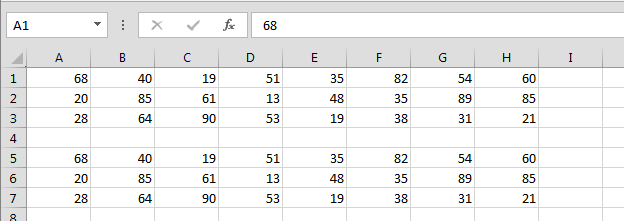# Change a Row or Rows to values to values using VBA in Microsoft Excel

We write the VBA coding to allow the Excel to do the repetitive work automatically within less time. In this chapter you will learn about how to change a row(s) to value(s) using VBA in Microsoft Excel.

Let’s take an example and understand how to write the VBA code for change a row(s) to value(s) using VBA.

How to change rows value to rows value?

We have data in Excel, in which we want the change the 1st rows value to 2nd rows value.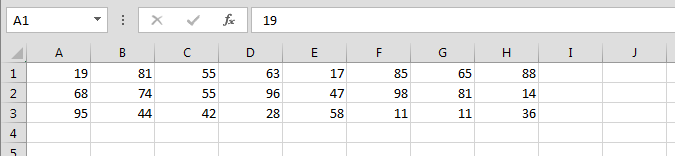• Press Alt+F11 key to open the Visual Basic Application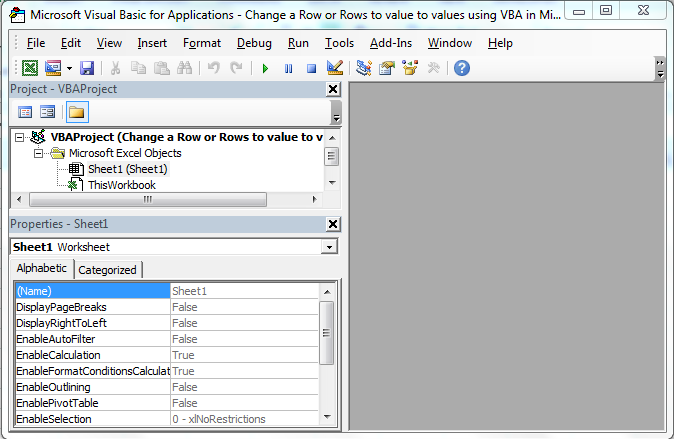• In VBAProject Double click on Sheet 1
• Enter the below given VBA Code
```Sub Values_6()

Rows("1").Value = Rows("2").Value

End Sub
```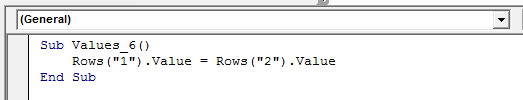• To run the code press F5 key
• 1st Row value will get update in the 2nd row value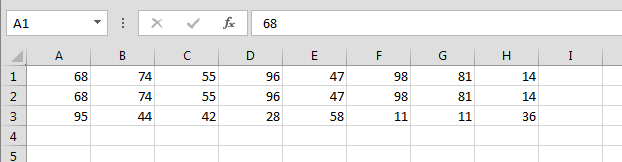How the change the multiple row values from other multiple row values?

We have 2 groups of row values, we want to change the 1st group value with the 2nd group value.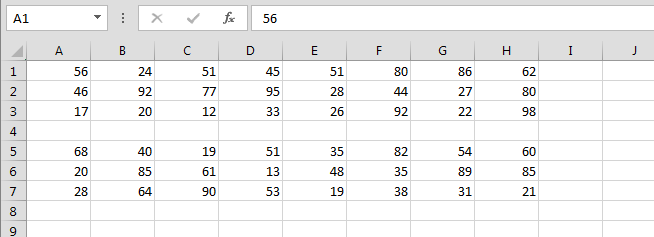To change the value follow below given steps and code:-

• Open the Visual Basic Application
• Enter the code below:-

```Sub Values_7()

Rows("1:3").Value = Rows("5:7").Value

End Sub
```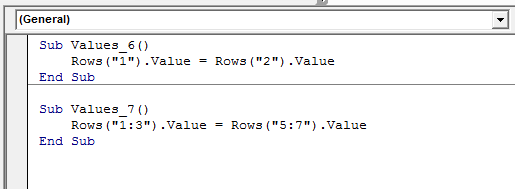• Run the code by pressing the F5
• Row Values will get update